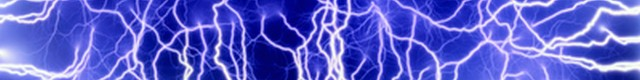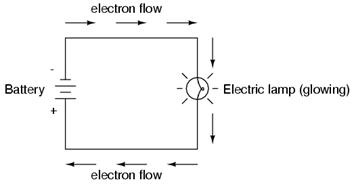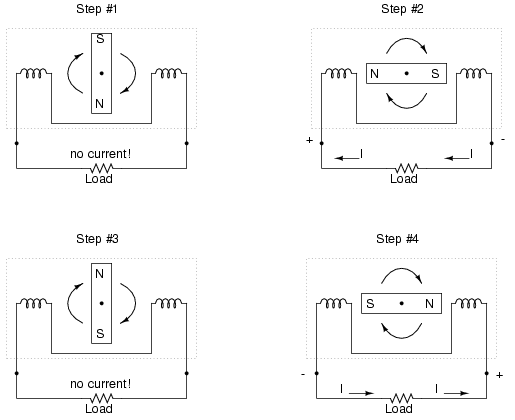# Electricity## Electricity

An electric charge exists whenever atoms gain or lose electrons. This is so because protons and electrons are attracted to each other, and in each of these states electrons want to move about trying to find and join atoms with extra protons. This electron movement or potential movement is what creates an electric charge.

There are two states for the condition of an electric charge.

The first is called static electricity whereby extra electrons build up on the surface of a material and there is no net flow of electrons into or away from the surface. When this negative type of charge comes close to a surface with a neutral or positive charge there is a quick discharge (neutralization) of the charge as the electrons jump to the other surface. See articles below.

http://science.howstuffworks.com/electricity-info.htm

http://en.wikipedia.org/wiki/Static_electricity

The second type of charge is called an electrical current (current electricity) where the extra electrons are moving unidirectionally from the negative charge to the positive charge. This happens when free electrons are attracted to an area where there are more protons than electrons and there is a channel for them to move there.

Creating electricity depends on the material (matter) involved. Basic classifications include conductor materials, semiconductor materials and insulator materials. Metals typically have more electrons than protons and hold on to their extra electrons more loosely, as such they are good conductors because electrons can move about more freely. Non-metals, e.g., wood, cloth, rubber, dry air, typically have more protons than electrons and hold on to their electrons more tightly, as such they are considered insulators because the electrons do not want to move easily. Semiconductor materials, such as Silicon (Si) and germanium (Ge), are in between and are useful for carrying a charge between conductor and insulator materials.

Direct Current vs Alternating Current Electricity

In today’s world the electrical current can be used in two ways. The first is called Direct Current electricity (DC) and the second is called Alternating Current electricity (AC).

Direct Current

Direct Current electricity is when an electrical current moves continuously in one direction. This direction involves electrons moving from a negatively charged area, which has extra electrons, to a positively charged area, which has extra protons.

DC current is what takes place when using batteries where electrons flow from a negative charge to a positive charge.Alternating Current

Alternating Current electricity involves a system that alternates the direction of electron flow back and forth by alternating the polarity. This is achieved by using a device called an armature, as part of an alternator, that creates reversing magnetic fields so that electrons will flow toward the positive field as it switches from one side of the system to the other.Volts, Amps, Ohms and Watts

http://science.howstuffworks.com/question501.htm

Volts, Amps, Watts and Ohms are the units used to define the condition of an electrical current. The most common way to communicate this is to use the analogy of water flowing through a hose, where flowing water is like flowing electrons. The water pressure is like volts, the rate of flow (current) is like amps, the resistance (size of hose) is like ohms, and the overall power, i.e., pressure x rate of flow, which in electricity is the volts x amps, is watts.

Ohms or resistance needs special clarification because the hose size comparison is incomplete. In a water hose the resistance depends on the size of the hose, whereas in electricity resistance depends on the both the size and type of material used, e.g., aluminum has a higher level of resistance than silver or copper. A thin copper wire has higher resistance than a thick copper wire.

http://en.wikipedia.org/wiki/Electrical_conductivity#Classification_of_materials_by_conductivity

There is debate about how to define electricity. See the following article:

http://www.eskimo.com/~billb/miscon/curstat.html